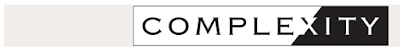## 30/04/2016

### A New Law for Complex Systems! Past the Power Law: The Limiting Law of Restricted Diversity and the 60/40 Rule

Over the past year we have worked on a series of articles which seek to provide a new way to measure the distribution of diversity in complex systems.  The result is a new way to measure complexity, called case-based entropy.  We published an article on this measure in Physica A.

In turn, we used this new measure to uncover a new limiting law that we found hidden in plain sight in a wide variety of skewed-right complex systems.  We call this limiting law restricted diversity, arguing that this law governs the distribution of diversity in skewed-right complex systems the same way the law of central tendency governs the distribution of diversity in normal distributions.

Even more compelling, restricted diversity can be rigorously measured in ways that far exceed the limits of the power law, revealing a 60/40 rule for skewed-right complex systems.  We just published these second two insights in a article in Complexity.

Below are the abstracts and links to the two papers:Past the power law: Complex systems and the limiting law of restricted diversity

Probability distributions have proven effective at modeling diversity in complex systems. The two most common are the Gaussian normal and skewed-right. While the mechanics of the former are well-known; the latter less so, given the significant limitations of the power-law. Moving past the power-law, we demonstrate that there exists, hidden-in-full-view, a limiting law governing the diversity of complexity in skewed-right systems; which can be measured using a case-based version C of Shannon entropy, resulting in a 60/40 rule. For our study, given the wide range of approaches to measuring complexity (i.e., descriptive, constructive, etc), we examined eight different systems, which varied significantly in scale and composition (from galaxies to genes). We found that skewed-right complex systems obey the law of restricted diversity; that is, when plotted for a variety of natural and human-made systems, as the diversity of complexity --> infinity (primarily in terms of the number of types; but also, secondarily, in terms of the frequency of cases) a limiting law of restricted diversity emerges, constraining the majority of cases to simpler types. Even more compelling, this limiting law obeys a scale-free 60/40 rule: when measured using C, 60%(or more) of the cases in these systems reside within the first 40% (or less) of the lower bound of equiprobable diversity types—with or without long-tail and whether or not the distribution fits a power-law. Furthermore, as an extension of the Pareto Principle, this lower bound accounts for only a small percentage of the total diversity; that is, while the top 20% of cases constitute a sizable percentage of the total diversity in a system, the bottom 60% are highly constrained. In short, as the central limit theorem governs the diversity of complexity in normal distributions, restricted diversity seems to govern the diversity of complexity in skewed-right distributions.

----------------------------------------------

# An entropy based measure for comparing distributions of complexity

This paper is part of a series addressing the empirical/statistical distribution of the diversity of complexity within and amongst complex systems. Here, we consider the problem of measuring the diversity of complexity in a system, given its ordered range of complexity types i and their probability of occurrence pi, with the understanding that larger values of i mean a higher degree of complexity. To address this problem, we introduce a new complexity measure called case-based entropy  Cc — a modification of the Shannon–Wiener entropy measure H. The utility of this measure is that, unlike current complexity measures–which focus on the macroscopic complexity of a single system–Cc can be used to empirically identify and measure the distribution of the diversity of complexity within and across multiple natural and human-made systems, as well as the diversity contribution of complexity of any part of a system, relative to the total range of ordered complexity types.